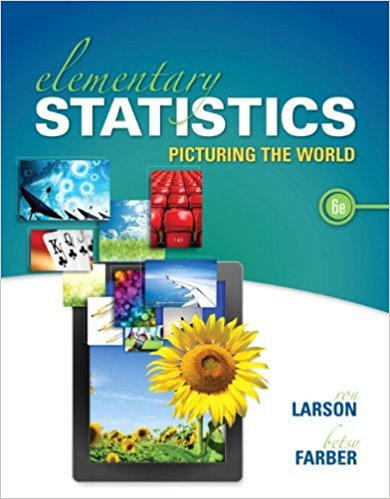×
Get Full Access to Elementary Statistics: Picturing The World - 6 Edition - Chapter 4.2 - Problem 16e
Get Full Access to Elementary Statistics: Picturing The World - 6 Edition - Chapter 4.2 - Problem 16e

×

# Solved: Finding Binomial Probabilities In Exercise, findISBN: 9780321911216 66

## Solution for problem 16E Chapter 4.2

Elementary Statistics: Picturing the World | 6th Edition

• Textbook Solutions
• 2901 Step-by-step solutions solved by professors and subject experts
• Get 24/7 help from StudySoup virtual teaching assistantsElementary Statistics: Picturing the World | 6th Edition

4 5 1 407 Reviews
26
4
Problem 16E

Problem 16E

Finding Binomial Probabilities In Exercise, find the indicated probabilities. If convenient, use technology or Table 2 in Appendix B to find the probabilities.

Childhood Obesity Thirty-nine percent of U.S. adults think that the government should help fight childhood obesity. You randomly select six U.S. adults. Find the probability that the number of U.S. adults who think that the government should help fight childhood obesity is (a) exactly two, (b) at least four, and (c) less than three.

Step-by-Step Solution:
Step 1 of 3

Solution 16E

Step1 of 4:

From the given problem we have an experiment, In that 39% of U.S. adults think that the government should help fight childhood obesity. Also, we have n = 6.

Here our goal is:

a). We need to find the probability of getting exactly two U.S. adults think that the government should help fight childhood obesity.

b). We need to find the probability of getting at least four U.S. adults think that the government should help fight childhood obesity.

c). We need to find the probability of getting less than three U.S. adults think that the government should help fight childhood obesity.

Step2 of 4:

a).

Let us consider a random variable ‘x’ it presents the number of U.S. adults think that the government should help fight childhood obesity. Let us assume that ‘x’ follows the Binomial distribution with parameters ‘n and p’. That is:And we know that the probability mass function of the Binomial distribution is:Then, The probability of getting exactly two U.S. adults think that the government should help fight childhood obesity is:Therefore, P(X = 2) = 0.3158.

Step3 of 4:

b).

The probability of getting at least four U.S. adults think that the government should help fight childhood obesity is:Where,is obtained from excel by using the function:

“=Binomdist(x, n, p, false)”.

 X0 0.051520374 1 0.197635534 2 0.315892862 3 0.269285719 Total 0.83433449

Now,Therefore,Step4 of 4:

c).

The probability of getting less than three U.S. adults think that the government should help fight childhood obesity is:Where,is obtained from excel by using the function:

“=Binomdist(x, n, p, false)”.

 X0 0.000262144 1 0.003538944 2 0.021233664 Total 0.5650

Therefore,Step 2 of 3

Step 3 of 3

##### ISBN: 9780321911216

Unlock Textbook Solution